# What is Pressure?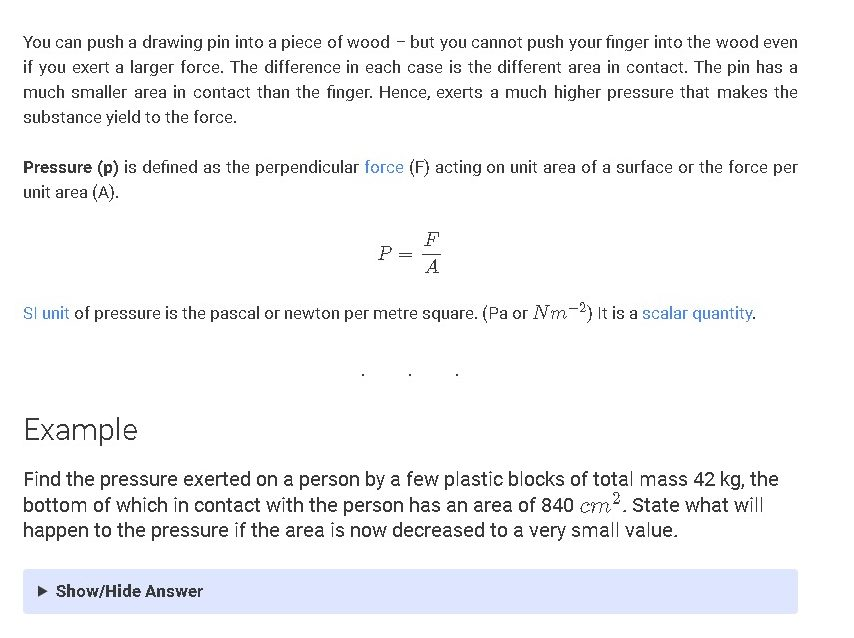You can insert a drawing pin into a piece of wood-but even though you have more leverage you can’t press your finger through the wood. The distinction being the particular interaction region of each situation. The pin is much smaller than the finger in touch. Therefore, the pressure exerted is much higher that gives the material power.

Pressure (p) is classified as a perpendicular force (F) operating on the surface area of the unit or intensity per area of unit (A).

ntent -->

SI unit of pressure is the pascal or newton per meter square. (Pa or Nm−2Nm−2) It is a scalar quantity.

## Example: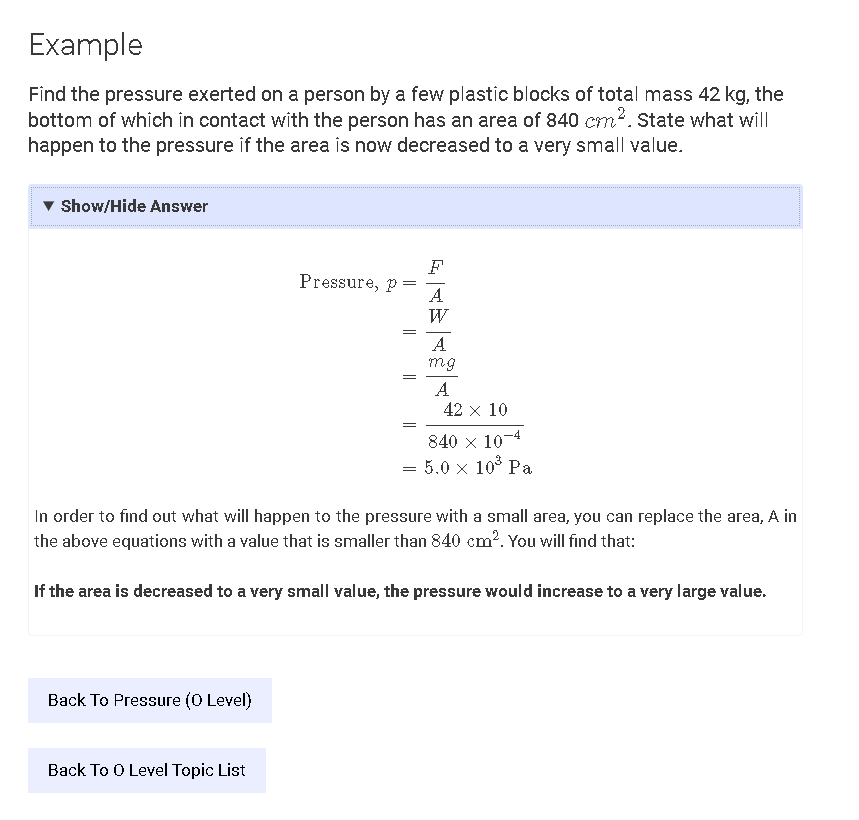Find out which of the plastic blocks with a total weight of 42 kg exert the pressure on a human, the bottom of which is 840cm2 in touch with the body. Tell if the strain would do if the area is now down to a very small value.

# Hydrostatic Pressure

Fluids and gases are known as fluids, and can quickly alter their types. A liquid is a moving material. In the figure above we see that the water from the right vessel goes above the water from the left vessel. The only distinction is the height of the water column between the two tanks. We realize, thus, that a deep (or height of a fluid column) of the fluid inside a body raises friction.

# Pressure Due to Fluid Column

Pressure due to a fluid column (p) = height of column (h) X density of the fluid (ρρ) X gravitational field strength (g). (The derivation of this formula can be found at the bottom of this post.)

p=ρghp=ρgh

In a fluid, if two points are separated by vertical height, their pressure difference is:

Δp=ρgΔhΔp=ρgΔh

From the formula, p=ρghp=ρgh, we can see that the pressure depends on the depth and density of the liquid and NOT on the cross-sectional area or volume of the liquid. Hence, the water pressure at the bottom of the container in the figure above will be the same throughout the container as the water level is the same! The shape of the container does NOT matter in the computation of the water pressure!

# Properties (Summary)

• The pressure is transmitted throughout the liquid
• Pressure acts in all directions
• All points at the same depth in a fluid are at the same pressure
• Pressure increases with depth
• The pressure is dependent not on the shape of the container but its depth.

# The pressure at Different Depths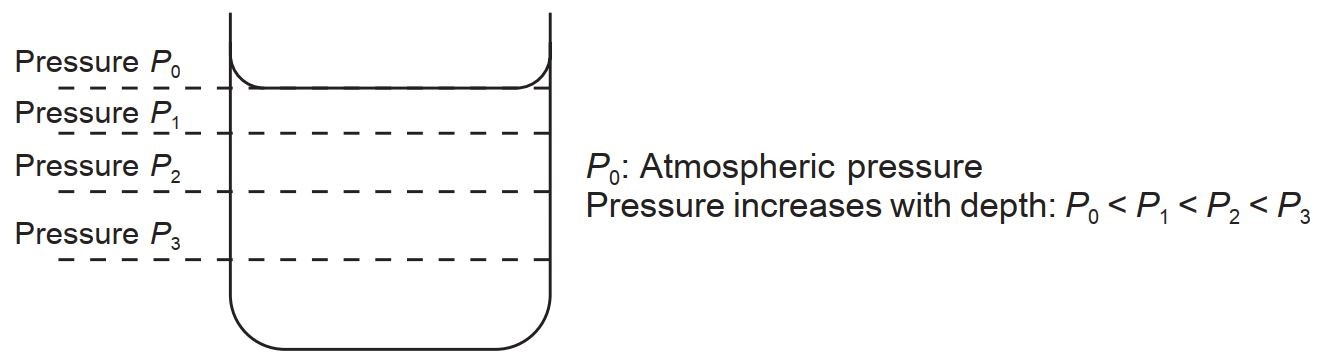An object immersed in a uniform liquid will experience a pressure which depends only on the height of the liquid above the object.

## Hence, consider the picture below.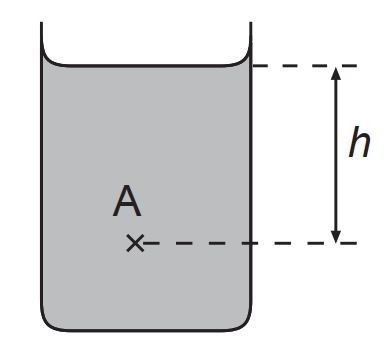The pressure at point A=P0+ρghPressure at point A=P0+ρgh,

where P0P0 is the atmospheric pressure

# A simple derivation of the formula for hydrostatic pressure (p=ρghp=ρgh):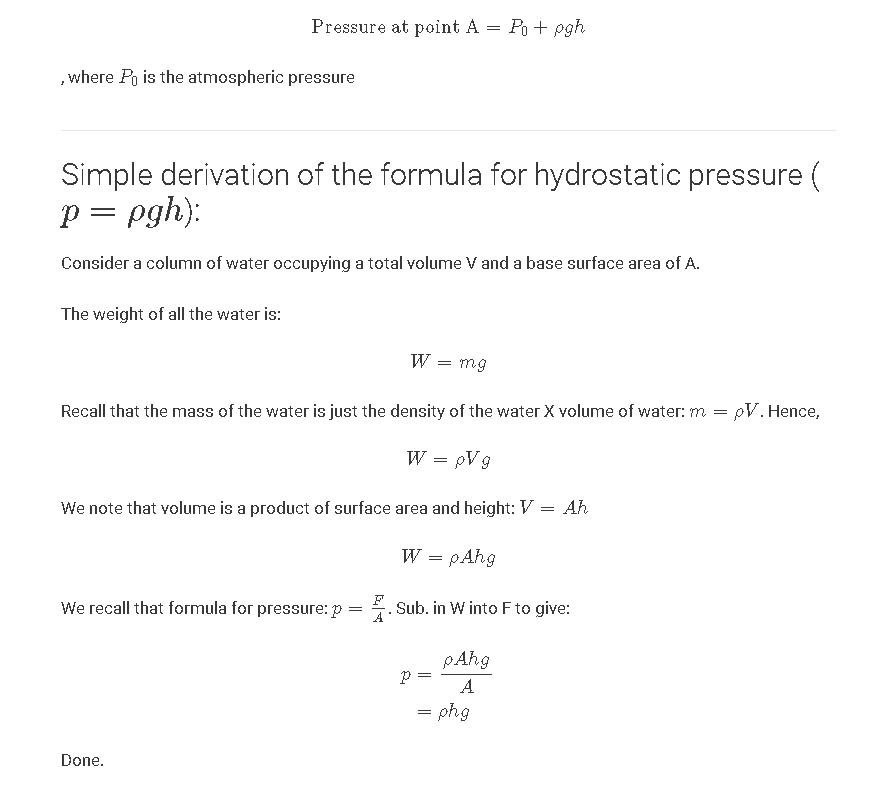# Atmospheric Pressure

Air is a fluid. We are living at the bottom of a “sea” of air called the atmosphere. The weight of the Earth’s atmosphere pushing down on each unit area of Earth’s surface constitutes the atmospheric pressure.

## Origin of Atmospheric Pressure

There is atmospheric friction due to the energetic air molecules’ molecular bombardment. A vast amount of air molecules travel at high speeds under natural conditions.

This vast number of air molecules interfere regularly with the container walls. The air molecules are rebounding from the wall and the rebound forces are exerting a strain on the wall.

The pressure on the wall is considered the air pressure on the wall by the air molecules. More information is available in the Three Matter States and the sub-topics below.

## Atmospheric Pressure at Sea Level

The pressure exerted by this layer of air at sea level is 1.013×105Pa1.013×105Pa. This value is referred to as one atmosphere and is equivalent to placing 1 kg weight on an area of 1 cm21 cm2. The pressure at higher altitudes is lower.

# Barometer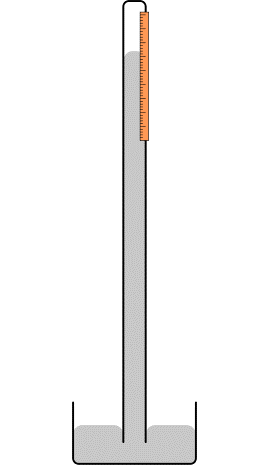## Mercury barometer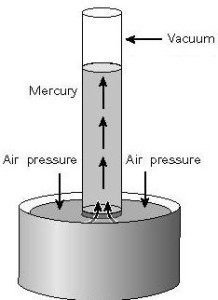A barometer is a basic method for air pressure assessment.

You can make a barometer by filling a wide glass tube with mercury and then twisting it in a mercury bath as shown. The region on top of the tube is a vacuum and the mercury column is not pressurized.

The atmosphere forces the mercury bath, forcing the mercury up the tube. That is why,

Pressure compared to a column of mercury = Pressure due to the pressure of the atmosphere by a column of mercury

The mercury column’s vertical height provides the requisite ambient pressure.

We know that from Hydrostatic Pressure:

Pair = column of mercury = pair = column of mercury > hp

, where h is the column height of mercury, μl is the mercury density, g is the acceleration of gravity.

Mercury Column Duration at 1 atmosphere

We can compute the length of the mercury column if 1 atm (1.013×105 Pa1.013×105 Pa) of atmospheric pressure is acting on the mercury bath. The computation is as follows: (Density of mercury is 13.6×103kg m−313.6×103kg m−3)

p1 atm=ρgh1.013×105=13.6×103×9.8×hh=0.760 mh=76 cmp1 atm=ρgh1.013×105=13.6×103×9.8×hh=0.760 mh=76 cm

## Barometers of Different Heights & Sizes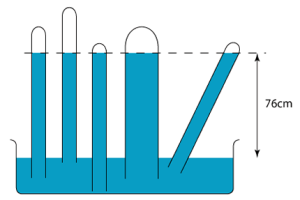The figure above shows the barometers of different heights and sizes. Since the principle behind the barometer is the phenomenon of hydrostatic pressure, we know that only the height of the fluid column is important in the determination of the pressure at the bottom. This means that the height, h in the above figure will remain unchanged if:

• the glass tube is lifted slightly from the dish
• the glass tube is lowered further into the dish
• the diameter of the glass tube increases
• the glass tube is tilted
• the quantity of mercury in the dish is increased.
• Pressures at Different Heights of a Barometer

Let’s consider the figure above. We have:

• The pressure at PaPais zero. (It is a vacuum in the air gap.)
• The pressure at PbPbis due to 26 cm of mercury.
• The pressure at Pepeis due to 76 cm of mercury.
• The pressure at PfPfis due to 84 cm of mercury.
• The pressure at PbPband PcPc is the same. Similarly, the pressure at PdPd and PePe is the same. This is because the points are at the same level.

# Characteristics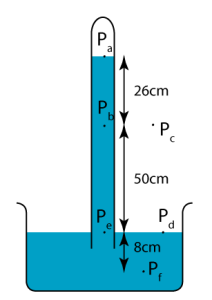Standard Atmosphere is the mean atmospheric pressure naturally existing at sea level on the surface of the Earth. It is equivalent to the pressure exerted by a vertical column of mercury (as in a barometer) 760 mm high or 101,325 Pa.

If the mercury is replaced by water, the vertical column of water equivalent to the atmospheric pressure is approximately 10 m.

The atmosphere pressure does vary from day to day and place to place.

# Manometer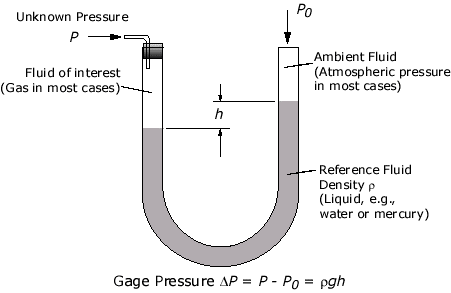A differential manometer is a simple instrument for comparing pressures, usually by the difference in height of two liquid columns.

The simplest of such an instrument is a U-tube containing some liquid, usually mercury, water, or oil.

The pressure exerted by a confined gas changes the levels of the mercury in the manometer. The total pressure of a gas or liquid is the total pressure it exerts, including the effect of atmospheric pressure. (patmpatm).

## Changes To Liquid Levels In Manometer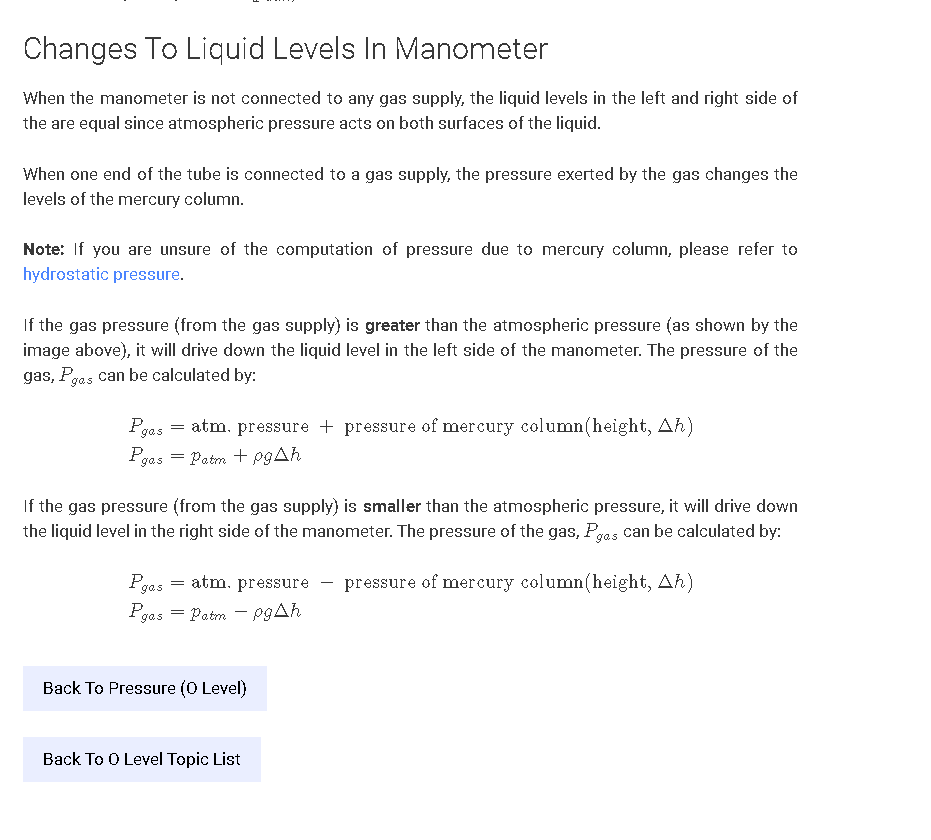When the manometer is not connected to any gas supply, the liquid levels in the left and right sides of the are equal since atmospheric pressure acts on both surfaces of the liquid.

When one end of the tube is connected to a gas supply, the pressure exerted by the gas changes the levels of the mercury column.

## Note:

If you are unsure of the computation of pressure due to the mercury column, please refer to hydrostatic pressure.

If the gas pressure (from the gas supply) is greater than the atmospheric pressure (as shown by the image above), it will drive down the liquid level on the left side of the manometer. The pressure of the gas, PgasPgas can be calculated by:

#### Related Posts